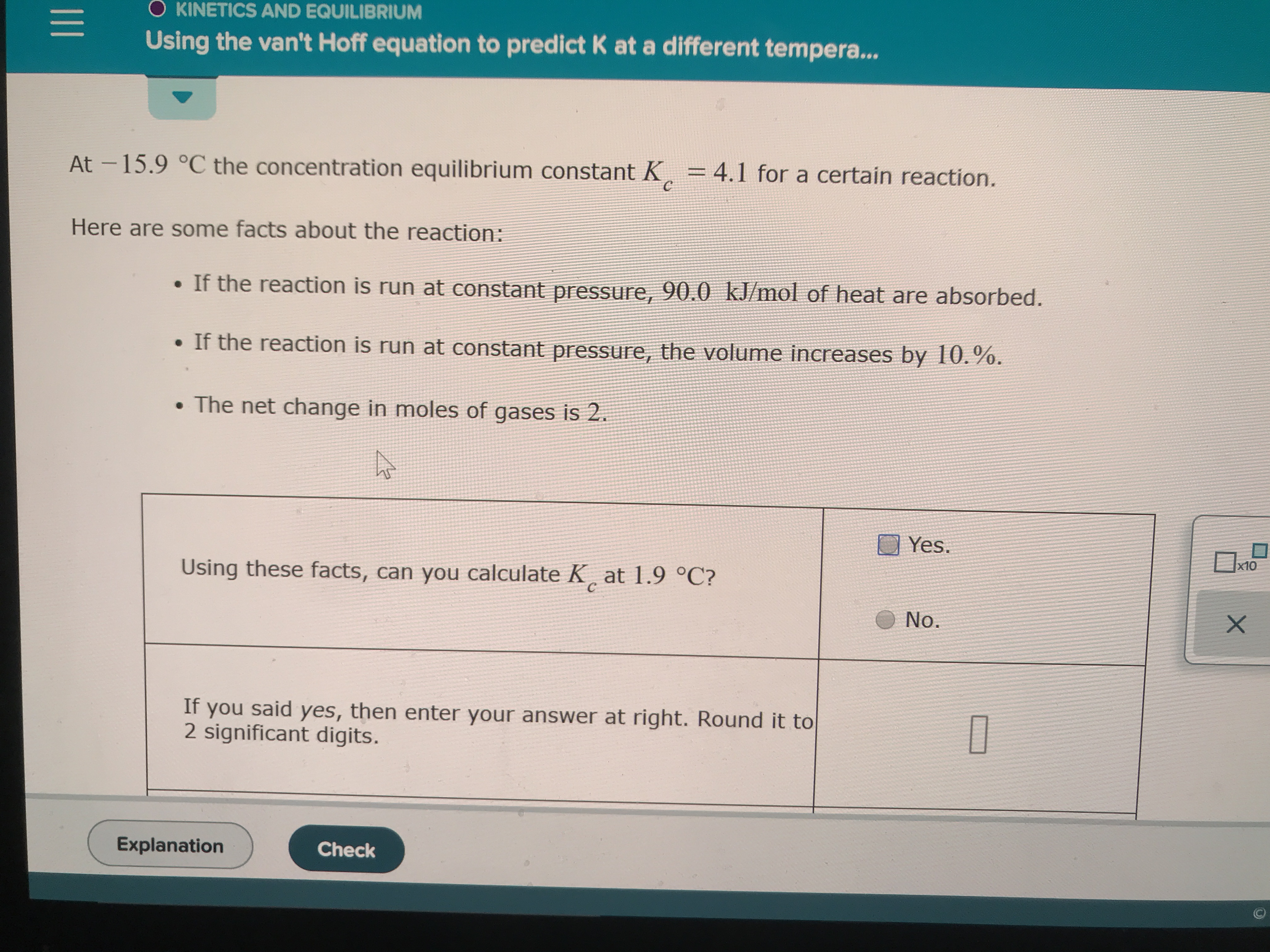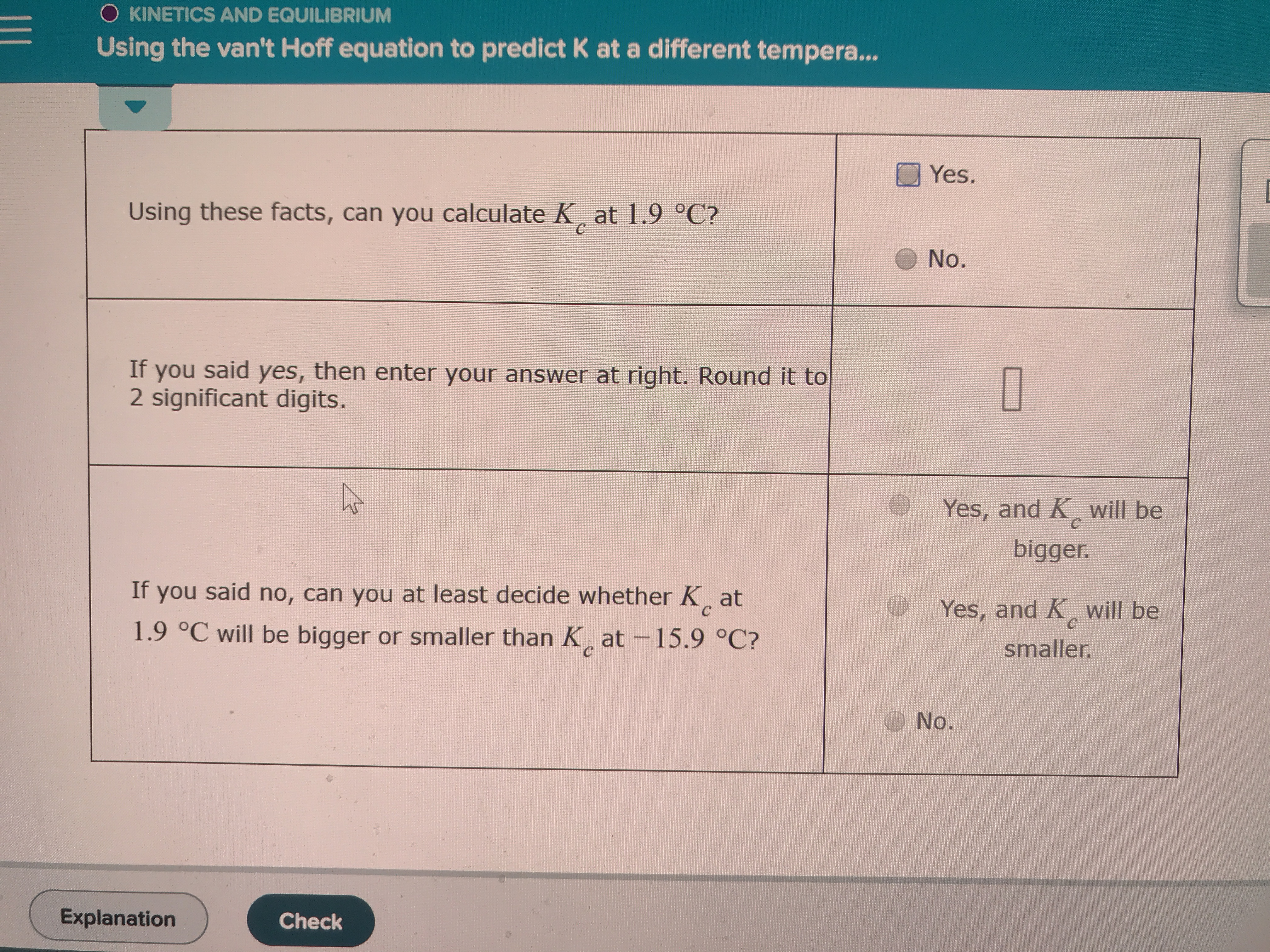# O KINETICS AND EQUILIBRIUMUsing the van't Hoff equation to predict K ata different tempera...At-15.9 °C the concentration equilibrium constant K= 4.1 for a certain reaction.Here are some facts about the reaction:If the reaction is run at constant pressure, 90.0 kJ/mol of heat are absorbed.. If the reaction is run at constant pressure, the volume increases by 10.%.The net change in moles of gases is 2.Yesx10Using these facts, can you calculate K at 1.9 °C?CNo.XIf you said yes, then enter your answer at right. Round it to2 significant digits.ExplanationCheck O KINETICS AND EQUILIBRIUMUsing the van't Hoff equation to predict K at a different tempera...Yes.Using these facts, can you calculate K at 1.9 °C?No.If you said yes, then enter your answer at right. Round it to2 significant digits.Yes, and K will bebiggerIf you said no, can you at least decide whether K atYes, and K will beс1.9 °C will be bigger or smaller than K at-15.9 °C?smaller.СNo.ExplanationCheck

Question
25 viewshelp_outlineImage TranscriptioncloseO KINETICS AND EQUILIBRIUM Using the van't Hoff equation to predict K ata different tempera... At-15.9 °C the concentration equilibrium constant K = 4.1 for a certain reaction. Here are some facts about the reaction: If the reaction is run at constant pressure, 90.0 kJ/mol of heat are absorbed. . If the reaction is run at constant pressure, the volume increases by 10.%. The net change in moles of gases is 2. Yes x10 Using these facts, can you calculate K at 1.9 °C? C No. X If you said yes, then enter your answer at right. Round it to 2 significant digits. Explanation Check fullscreenhelp_outlineImage TranscriptioncloseO KINETICS AND EQUILIBRIUM Using the van't Hoff equation to predict K at a different tempera... Yes. Using these facts, can you calculate K at 1.9 °C? No. If you said yes, then enter your answer at right. Round it to 2 significant digits. Yes, and K will be bigger If you said no, can you at least decide whether K at Yes, and K will be с 1.9 °C will be bigger or smaller than K at-15.9 °C? smaller. С No. Explanation Check fullscreen
check_circle

Step 1

The temperature of -15.90C is equivalent to 257.25 K and the temperature of 1.90C is equivalent to 275.05 K. The absorbed heat of 90.0 kJ/mol is equivalent to 90.0×103 J/mol. Heat absorbed at constant pressure is defined as the enthalpy change.

Step 2

On the basis of given facts, Kc at 275.05 K is calculated as shown in equation (1) where 254.25 K is the initial temperature, 275.05 K is the final temperature,...

### Want to see the full answer?

See Solution

#### Want to see this answer and more?

Solutions are written by subject experts who are available 24/7. Questions are typically answered within 1 hour.*

See Solution
*Response times may vary by subject and question.
Tagged in

### Equilibrium Concepts